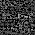11. what is waveguide cutoff frequency , guide wavelength , phase velocity , group velocity , propagation constant

11. what is waveguide cutoff frequency , guide wavelength , phase velocity , group velocity , propagation constant

Lets explain cutoff frequency , guide wavelength , group velocity , phase velocity and propagation constant of a waveguide.

Cutoff frequency :

Cutoff frequency is the frequency below which attenuation occurs and above which propagation takes place.Each mode have a specific cutoff frequency.

For TEmn modes the cutoff frequency is given by
$f_{c}=\frac{1}{2\sqrt{\mu \varepsilon}}\sqrt{{(\frac{m}{a})}^{2}+{(\frac{n}{b})}^{2}}$
Guide Wavelength :

It is the distance traveled by the wave in order to undergo a phase shift of 2π radians.
It is related to propagation constant β as

$\lambda_{b}=\frac{2\pi}{\beta}$
Wavelength in waveguide is different from wavelength in free space.

Relation between cutoff frequency and guide wavelength :

The relationship between two is as follows
$\frac{1}{\lambda_{0}^{2}}=\frac{1}{\lambda_{g}^{2}}+\frac{1}{\lambda_{c}^{2}}$
Also it can be written as
$\lambda_{g}=\frac{\lambda_{0}}{\sqrt{1-\Big(\frac{\lambda_{0}}{\lambda_{c}}}\Big)^{2}}$
When ⋋0 ≪ ⋋c , then ⋋g= ⋋0
When ⋋0 = ⋋c , then ⋋g becomes ∞
When ⋋0 > ⋋c , then ⋋g becomes imaginary ,that means no propagation in the waveguide

Where ⋋0 is the free space wavelength.

Phase velocity :

The phase velocity of a wave is the rate at which the phase of the wave propagates in space.
The phase velocity is given by
$v_{p}=\frac{\omega}{k}$
Where k = wave number

Also it is given as
$v_{p}=\frac{c}{\sqrt{1-\Big(\frac{\lambda_{0}}{\lambda_{c}}\Big)^{2}}}$

Group velocity :

If there is modulation in carrier, the modulation envelope travels at a velocity slower than the carrier. This velocity of the modulation envelope is called as group velocity.

Or in other words

The group velocity of a wave is the velocity with which the overall shape of the waves' amplitudes — known as the modulation or envelope of the wave — propagates through space.

It is given as
$v_{g}=\frac{\partial \omega}{\partial k}$
Also it is given as
$v_{g}=c\sqrt{1-\Big(\frac{\lambda_{0}}{\lambda_{c}}\Big)^{2}}$

Relationship between group velocity and phase velocity :
$v_{p}v_{g}=c^{2}$

Propagation constant :

The propagation constant of an electromagnetic wave is a measure of the change undergone by the amplitude of the wave as it propagates in a given direction

For a TEmn mode it is given by

$\beta=\sqrt{\mu\epsilon}\sqrt{\omega^{2}-\omega_c^2}$

1.Amazing!
This is a valuable content which i want to collect. I like it so much.
2.3.